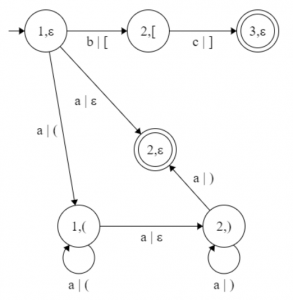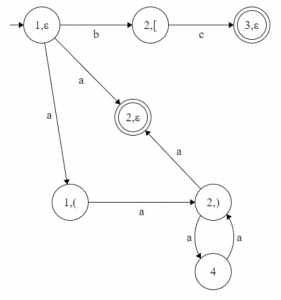# Hypothesis (language regularity) and algorithm (L-graph to NFA) in TOC

Prerequisite – Finite automata, L-graphs and what they represent
L-graphs can generate context sensitive languages, but it’s much harder to program a context sensitive language over programming a regular one. This is why I’ve came up with a hypothesis about what kind of L-graphs can generate a regular language. But first, I need to introduce you to what I call an iterating nest.

As you can remember a nest is a neutral path, whereandare cycles andpath is neutral. We will callan iterating nest, if,andpaths print the same string of symbols several times, to be more exactprints,prints,prints,andis a string of input symbols (better, if at least one of).
From this definition comes out the next hypothesis.

Hypothesis – If in a context free L-graph G all nests are iterating, then the language defined by this L-graph G, L(G), is regular.
If this hypothesis will be proven in the near future, it can change a lot in programming that will make creating new easy programming languages much easier than it already is. The hypothesis above leads to the next algorithm of converting context free L-graphs with iterating nests to an NFA.

Algorithm – Converting a context free L-graph with iterating complements to a corresponding NFA
Input – Context free L-graphwith iterating complements
Output –• Step-1: Languages of the L-graph and NFA must be the same, thusly, we won’t need a new alphabet. (Comment: we build context free L-graph G’’, which is equal to the start graph G’, with no conflicting nests)
• Step-2: Build Core(1, 1) for the graph G.
V’’ := {(v,) | vV ofcanon kCore(1, 1), vk}:= { arcs| start and final statesV’’}

For all kCore(1, 1):
Step 1’. v := 1st state of canon k..
V’’Step 2’.arc from statefollowed this arc into new state defined with following rules:, if the input bracket on this arc;, if the input bracket is an opening one;, if the input bracket is a closing bracket
v := 2nd state of canon k
V’’Step 3’. Repeat Step 2’, while there are still arcs in the canon.

• Step-3: Build Core(1, 2).
If the canon has 2 equal arcs in a row: the start state and the final state match; we add the arc from given state into itself, using this arc, to.
Add the remaining inarcs v – utoin the form of• Step-4:(Comment: following is an algorithm of converting context free L-graph G’’ into NFA G’)

• Step-5: Do the following to every iterating complementin G’’:
Add a new state v. Create a path that starts in state, equal to. From v intocreate the path, equal to. Delete cyclesand.

• Step-6: G’ = G’’, where arcs are not loaded with brackets.

So that every step above is clear I’m showing you the next example.Context free L-graph with iterating complements,
which determines theStart graph GCore(1, 1) = { 1 – a – 2 ; 1 – a, (1 – 1 – a – 2 – a, )1 – 2 ; 1 – b, (2 – 2 – c, )2 – 3 }
Core(1, 2) = Core(1, 1){ 1 – a, (1 – 1 – a, (1 – 1 – a – 2 – a, )1 – 2 – a, )1 – 2 }
Step 2: Step 1’ – Step 3’Intermediate graph G’’NFA G’

My Personal Notes arrow_drop_upCheck out this Author's contributed articles.

If you like GeeksforGeeks and would like to contribute, you can also write an article using contribute.geeksforgeeks.org or mail your article to contribute@geeksforgeeks.org. See your article appearing on the GeeksforGeeks main page and help other Geeks.

Please Improve this article if you find anything incorrect by clicking on the "Improve Article" button below.